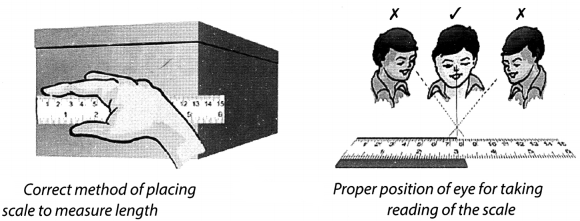On this page, you will find Motion and Measurement of Distances Class 6 Notes Science Chapter 10 Pdf free download. CBSE NCERT Class 6 Science Notes Chapter 10 Motion and Measurement of Distances will seemingly help them to revise the important concepts in less time.

## CBSE Class 6 Science Chapter 10 Notes Motion and Measurement of Distances

### Motion and Measurement of Distances Class 6 Notes Understanding the Lesson

1. In prehistoric times, the humans did not have means of transport. They moved around on foot.

2. Invention of wheels made a great change in modes of transport.

3. Animals were used to pull vehicles that moved on wheels.

4. Until the beginning of the 19th century, people still depended on animal power to transport them from place to place.

5. The invention of steam engine introduced a new source of power.

6. In our day to day life, measurement of distance or length is a very common activity.

7. Measurement involves comparison of an unknown quantity with some known fixed quantity of the same kind. This known fixed quantity is called a

8. Each measurement consists of a number describing the numerical value of the measure and the unit in which the quantity is measured.

9. While measuring things, accuracy is needed. A variety of units can be used for this purpose.10. A unit of measurement which has fixed value and which does not change from person to person or place to place, is called standard unit of measurement.

11. To make accurate measurement, we need a proper measuring device.

12. Some commonly used length-measuring devices are:

(a) Ruler or metre scale
(b) Measuring tape13. While measuring length using a metre scale or a ruler, we must follow carefully the steps given below:

(a) The ruler should be kept along the length of the object so that the zero mark on the ruler coincides with the one end of the object as shown in figure.
(b) While taking the measurement, the eyes should be correctly positioned.(c) If the edge of the ruler is worn out or broken, the measurement should be taken from any other full mark of the ruler.

14. For measuring a curved line, a string and a ruler is used.

15. The string is placed along a curved line and its ends are marked on the string.

16. The length of the string between the marked point is measured using a ruler.

17. When we look around, we find that some objects are at rest and some are moving or in motion.

18. The motion of all the objects are not of the same type.

19. Some of the important types of motion are:

• Rectilinear motion
• Circular motion
• Periodic motion

20. Some objects may have more than one type of motion at the same time.

### Class 6 Science Chapter 10 Notes Important Terms

Circular motion: When a body moves in a circular path, its motion is known as circular motion.

Distance: Measurement of gap between two points in certain units is called distance.

Measurement: The comparison of some unknown quantity with a fixed known quantity of the same kind is called measurement.

Motion: The state of objects in which they are moving, e., they are changing their place with time is called motion.

Periodic motion: The motion which repeats itself after regular intervals of time, is called periodic motion.

Rectilinear motion: When a body moves along a straight-line path, it is called rectilinear motion.

SI units: A unit of measurement which has fixed value and it does not change from person to person or place to place is called SI units.

Units of measurement: The fixed quantity with the help of which comparison of some unknown quantity is done is called units of measurement.